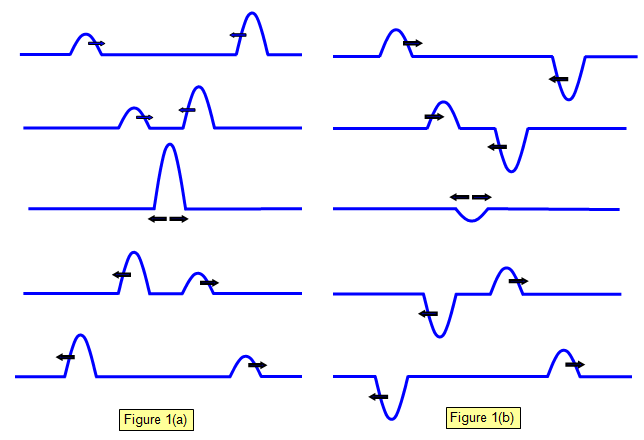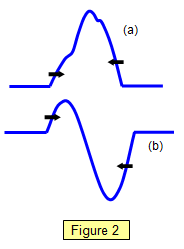The superposition of waves

When two waves overlap the resulting disturbance is simply the sum of the disturbances produced by each wave. Imagine two waves moving towards each other as shown in Figure 1. Figure 1(a) shows two pulses of the same phase while Figure 1(b) shows two pulses of opposite phase.Notice that the pulses move through each other, their final shape and amplitude being unchanged.With pulses of the same phase the pulses reinforce while those of opposite phase tend to cancel each other. If the pulses are of the same shape and amplitude they will completely cancel at one point as they pass through each other.

Figure two shows two pulses in the act of passing through each other. Figure 2(a) shows two pulses of the same sign while Figure 2(b) shows two pulses of opposite sign.

A VERSION IN WORD IS AVAILABLE ON THE SCHOOLPHYSICS USB